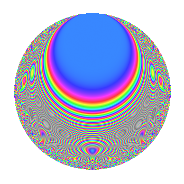# Properties

 Label 105.2.xLevel 105 Weight 2 Character orbit x Rep. character $$\chi_{105}(2,\cdot)$$ Character field $$\Q(\zeta_{12})$$ Dimension 48 Newform subspaces 1 Sturm bound 32 Trace bound 0

# Related objects

## Defining parameters

 Level: $$N$$ = $$105 = 3 \cdot 5 \cdot 7$$ Weight: $$k$$ = $$2$$ Character orbit: $$[\chi]$$ = 105.x (of order $$12$$ and degree $$4$$) Character conductor: $$\operatorname{cond}(\chi)$$ = $$105$$ Character field: $$\Q(\zeta_{12})$$ Newform subspaces: $$1$$ Sturm bound: $$32$$ Trace bound: $$0$$

## Dimensions

The following table gives the dimensions of various subspaces of $$M_{2}(105, [\chi])$$.

Total New Old
Modular forms 80 80 0
Cusp forms 48 48 0
Eisenstein series 32 32 0

## Trace form

 $$48q - 2q^{3} - 24q^{6} - 12q^{7} + O(q^{10})$$ $$48q - 2q^{3} - 24q^{6} - 12q^{7} - 8q^{10} - 10q^{12} - 16q^{13} + 4q^{15} - 8q^{16} + 14q^{18} - 28q^{21} - 8q^{22} + 4q^{25} + 40q^{27} - 60q^{28} + 40q^{30} - 24q^{31} - 4q^{33} + 8q^{36} + 4q^{37} - 16q^{40} + 14q^{42} + 16q^{43} + 40q^{45} - 32q^{46} + 44q^{48} + 8q^{51} + 36q^{52} - 40q^{55} - 88q^{57} + 56q^{58} - 50q^{60} - 8q^{61} + 44q^{63} + 76q^{66} + 12q^{67} + 140q^{70} - 34q^{72} + 52q^{73} + 6q^{75} + 64q^{76} - 120q^{78} + 20q^{81} + 104q^{82} - 24q^{85} - 46q^{87} - 84q^{90} + 72q^{91} - 44q^{93} + 12q^{96} - 120q^{97} + O(q^{100})$$

## Decomposition of $$S_{2}^{\mathrm{new}}(105, [\chi])$$ into newform subspaces

Label Dim. $$A$$ Field CM Traces $q$-expansion
$$a_2$$ $$a_3$$ $$a_5$$ $$a_7$$
105.2.x.a $$48$$ $$0.838$$ None $$0$$ $$-2$$ $$0$$ $$-12$$

## Hecke Characteristic Polynomials

There are no characteristic polynomials of Hecke operators in the database